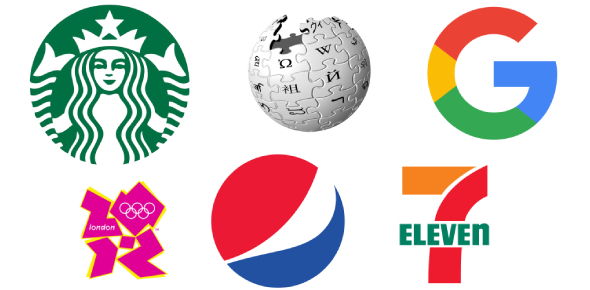# Science Quiz: Physics And Chemistry! Trivia

20 Questions | Total Attempts: 13SettingsCreate your own QuizAre you thirsty for knowledge? Do you want to unravel the scientific mysteries? If you want, then take this quiz to test your understanding of physics and chemistry. This quiz contains questions from all the topics. Learn and assess your science concepts. Let's give it a try. All the best!

• 1.
The strain is defined as the ratio of:
• A.

Change in length to the original length

• B.

Change in volume to the original volume

• C.

Change in cross-sectional area to original cross-sectional area

• D.

Any one of the above

• 2.
The unit of Young’s modulus is:
• A.

Mm/mm

• B.

Kg/cm

• C.

Kg

• D.

Kg/cm2

• 3.
The impact strength of a material is an index of its:
• A.

Toughness

• B.

tensile strength

• C.

The capability of being cold worked

• D.

Hardness

• 4.
Several forces acting at a point will be in equilibrium if:
• A.

Some of the forces are zero

• B.

The algebraic sum of the forces is zero

• C.

Some of the resolved parts in any two perpendicular directions are both zero

• D.

None of the above

• 5.
Two forces of 6 and 8 kg act at right angles to each other, the resultant force will be (use parallelogram)
• A.

10

• B.

100

• C.

58

• D.

48

• 6.
The flow of the refrigerant in a refrigeration cycle is controlled by:
• A.

Compressor

• B.

Condenser

• C.

Evaporator

• D.

Expansion valve

• 7.
Sub-cooling is
• A.

Cooling a part of the liquid

• B.

Differential cooling of a liquid

• C.

Removing latent heat from a gas

• D.

Removing sensible heat from a liquid

• 8.
A flange coupling is
• A.

Used for non-collinear shafts

• B.

Used for collinear shafts

• C.

Flexible

• D.

Used only on small shafts rotating at slow speeds

• 9.
In the case of a knuckle joint the pin is likely to fail in
• A.

Compression

• B.

Crushing

• C.

Tension

• D.

Double shear

• 10.
The rivet diameter should be:
• A.

Half the plate thickness

• B.

Less than the plate thickness

• C.

Equal to the strap thickness

• D.

More than the plate thickness

• 11.
The accuracy depends upon
• A.

Precision of instrument

• B.

Precision of method

• C.

Good planning

• D.

All of the above

• 12.
Which of the following is an indirect pressure measuring device?
• A.

Bourdon tube

• B.

Flat diaphragm

• C.

Ionization gauge

• D.

Manometer

• 13.
In a car the fuel level is measured by:
• A.

Electronic transducer

• B.

Float method

• C.

Dip stick

• D.

Any of the above

• 14.
Which motorcycle has a maximum power rating?
• A.

Jawa

• B.

Rajdoot

• C.

Yezdi

• D.

Yamaha

• 15.
In a four-stroke engine, each cylinder has:
• A.

One valve

• B.

Two valves

• C.

Three valves

• D.

Four valves

• 16.
The purpose of the dynamo in an automobile is to:
• A.

Supply electric power

• B.

Convert mechanical energy into electrical energy

• C.

Act as a reservoir of electrical energy

• D.

Continually recharge the battery

• 17.
Solar cells are made of:
• A.

Silica

• B.

Steel

• C.

Sulphur

• D.

Antimony

• 18.
One calorie is the same as:
• A.

1 dyne

• B.

1 dyne-sec

• C.

1 watt

• D.

4.18 joules

• 19.
Metals are good conductors of heat because:
• A.

They contain free electrons

• B.

Their atoms are relatively far apart

• C.

Their atoms collide frequently

• D.

They have reflecting surfaces

• 20.
Which one of the following will have the least value of thermal conductivity?
• A.

Copper

• B.

Silver

• C.

Glass

• D.

Air

Related TopicsBack to top
×

Wait!
Here's an interesting quiz for you.